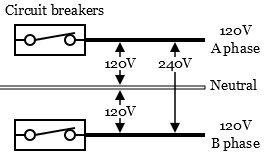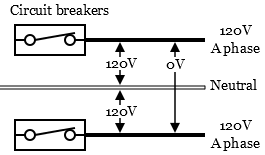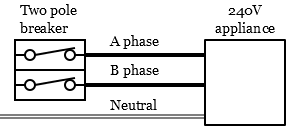﻿ Electrical Voltages - Electrical 101Question or Comment?

Residential voltage in the USA and Canada is 120 / 240 volts AC. Power enters the dwelling’s main electrical panel from a power company transformer as two 120 volt lines with phases that are 180 degrees apart. 120 and 240 volts (along with neutral and ground) is then distributed to outlet boxes (switch, receptacle, light fixture, etc) throughout the dwelling.

## Nominal Voltage

110, 115, 120, 125, 130, 220, 230, 240, 250 volts, what are all these different voltages?

Nominal voltage - 120 volts & 240 volts are the standards to designate a voltage class for residential buildings. All other voltages are high or low voltage ratings of light bulbs, appliances, electronics etc.

Higher voltage ratings of 125, 130, 230, and 250 volts are for switches, receptacles, bulbs, and certain loads. These ratings indicate the upper limit of voltage for a device or load to operate properly under normal conditions.

Lower voltage ratings of 110 , 115, and 220 volts are for loads (appliances, motors, etc). These ratings indicate the lower limit of voltage for proper operation under normal conditions.

## 240 Volts AC

Residential electric ranges, electric dryers and central air conditioners usually need 240 volts to operate. 240 volts is achieved when combining two sources of 120 volts of different phases (A and B phases). B phase current flows in the opposite direction as A phase. When A phase voltage peaks at +170 volts, B phase is at -170 volts.

# Electrical Voltages

### 240 Volts Power Wiring Diagrams

The potential difference (voltage) between A and B phase 120 volts is 240 volts. The potential difference of two 120 volts lines at the same phase is 0 volts. A and B phase voltage is needed to supply 240 volts to a load.

Voltage between A and B phase is 240 VoltsVoltage between A and A phase is 0 Volts### 240 Volts Appliance Wiring Diagram120 volts B Phase Sine Wave Diagram### 120 volts A Phase Sine Wave Diagram

This is a wiring diagram of a 240 volts circuit for an appliance. A two pole breaker supplies 120 volts A  and B to get 240 volts.